線性代數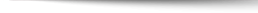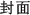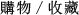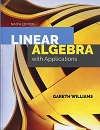Linear Algebra: with Applications 9/e

+作者：

Williams

+年份：
2019 年9 版
+ISBN：
9781284120097
+書號：
MA0424HC
+規格：

+頁數：
616
+出版商：
Jones & Bartlett

\$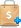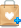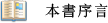Linear Algebra with Applications, Ninth Edition is designed for the introductory course in linear algebra for students within engineering, mathematics, business management, and physics. Updated to increase clarity and improve student learning, the author provides a flexible blend of theory and engaging applications.

The material in Linear Algebra with Applications, Ninth Edition is arranged into three parts that contain core and optional sections:
Part 1 introduces the basics, discussing systems of linear equations, vectors in Rn matrices, linear transformations, determinants, eigenvalues, and eigenspaces.
Part 2 builds on this material to discuss general vector spaces, and includes such topics as the Rank/Nullity Theorem, inner products and coordinate representation.
Part 3 completes the course with important ideas and methods in Numerical Linear Algebra including ill-conditioning, pivoting, LU decomposition and Singular Value Decomposition.

Throughout the text the author provides interesting applications, ranging from theoretical applications such as the use of linear algebra in differential equations, to many practical applications in the fields of electrical engineering, traffic analysis, relativity, history, and more.●Updated to simplify earlier material in the text while going into later, more advanced material in more depth
●Interesting applications, both theoretical and practical, engage and challenge students
●Carefully explained and illustrated examples highlight key concepts through the text
●A MATLAB manual, included as an appendix, consists of 31 sections that tie into course material
●Available with WebAssign Online Homework and Assessment with integrated eBook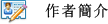Gareth Williams earned a B.S. and a Ph.D. in applied mathematics from the University of Wales, and did graduate work at Kings College University of London. He has taught mathematics at the University of Florida, the University of Denver, and Stetson University. He has been an exchange professor at the Paedagogische Hochschule, in Freiburg, Germany and has spent sabbaticals at the University of Wales. His mathematical interests include linear algebra, relativity, mathematical modeling, and the computer in mathematics education.

His publications include "Fine Topologies for Minkowski Space", Motions in Relativistic Space", "Mathematics in Archaeology", and books on Finite Mathematics, Calculus, and College Algebra. He is the co-developer (with Lisa Coulter) of the linear algebra software package, "The Linear Algebra Toolbox" for MATLAB. Dr. Williams is a member of The Mathematics Association of America.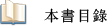Part  1  Linear Equations, Vectors, and Matrices
Chapter  1  Linear Equations and Vectors
Chapter  2  Matrices and Linear Transformations
Chapter  3  Determinants and Eigenvectors
Part  2  Vector Spaces
Chapter  4  General Vector Spaces
Chapter  5  Coordinate Representations
Chapter  6  Inner Product Spaces
Part  3  Numerical Linear Algebra
Chapter  7  Numerical Methods
Chapter  8  Linear Programming
Appendix  A  Cross Product
Appendix  B  Equations of Planes and Lines in Three-Space
Appendix  C  Graphing Calculator Manual
Appendix  D  MATLAB Manual